# Speed Control of DC Motor

## What is a DC Motor?

DC motor is an electrical machine that converts electrical energy to mechanical energy. It's called a DC motor because the power supply used is DC (direct current).

## Working of DC motor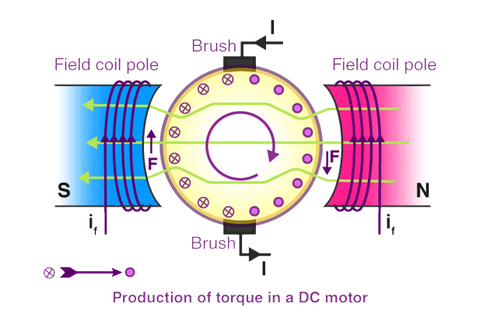DC motor works on the principle that when a current-carrying conductor is placed in a magnetic field it experiences a force which is given by Flemming’s Left-hand rule ( When you keep your forefinger, middle finger and thumb perpendicular to each other and forefinger represent the direction of the magnetic field, the middle finger represents the current flowing through the armature then the thumb represents the direction of the force generated on the armature coil.)
As the armature coil rotates it cuts the magnetic flux lines which result in a change in the magnetic field giving rise to back emf according to faradays law of electromagnetic induction. This induced emf is in the opposite direction of the applied voltage. This back emf makes the dc motor self-regulating. Example: When you increase the load on the motor, the speed of the motor gets reduced. This decreases the back emf and increases the current through armature thereby
increasing the torque to compensate for the applied load. Likewise, when you decrease the load, the speed of the motor increases. This increases the back emf and decreases the current through the armature by which the torque is reduced to make up for the load.

## Why speed control is necessary?

There are various applications in which speed control of the dc motor plays a crucial role.
Imagine you are in a car. To get a comfy ride in traffic, you will need to control the speed of the vehicle. Similarly in electronics, to control the movements of robots or drones so that they won't crash speed control is used. For drones, you get

The difference between speed control and speed regulation is that speed control is done automatically or manually whereas speed regulation is the measure of the regulation(maintaining) of the constant speed on changing load conditions at the motor shaft.

## Speed control methods of DC Motor

The speed of a dc motor is given by the following equation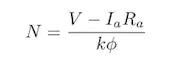N is the speed of the dc motor

V is the voltage across the armature

Ra is the armature resistance

Ia is the current through the armature

K is constant and ϕ is the flux

From the equation we can see that speed control of the dc motor can be achieved by changing flux which is done by changing field current (Flux control method), changing armature resistance(Armature control method) or voltage supply(Voltage control method). Let's see each one of them one by one

## How to control the speed of DC motor

### 1. Flux/Field control method

Here we change the magnetic flux to control the speed of the motor. To achieve this, we change the current through the field winding. So to do this we place a resistor in series with the field winding and vary this resistor to control the current through
which we control the field and then finally the speed of the dc motor. When resistance is set to a minimum, the current through the field winding increases, and thus flux also increases and speed decreases from the equation of speed and below equation.

ϕ = 𝐾fIf
Kf is the magnetic field design constant.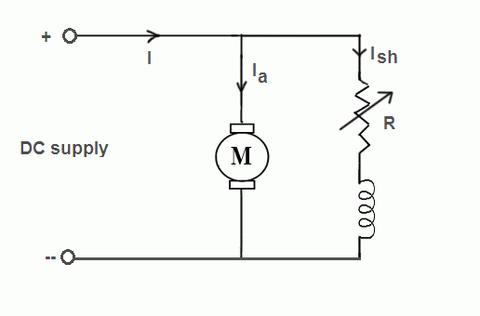### 2. Armature control method

In this method, a variable resistor is placed in series with the armature winding.
When there is a change in resistance, the voltage across the armature gets changed, and thus the speed. So, if the variable resistor’s resistance is decreased, the voltage drop across the armature winding is increased according to ohm’s law, and thus speed is increased. Similarly, when the variable resistor is increased, the voltage drop across the armature winding decreases and speed decreases.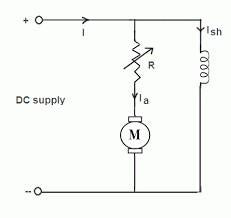### 3. Voltage control method

This method is the most extensively used method for controlling the speed of the motor. In this method, we change the supply voltage to the motor and vary its speed. So, increasing or decreasing the supply voltage to the motor will increase or decrease the speed of the motor respectively.
The voltage across the motor can be varied by applying a PWM(pulse width modulation) signal. This PWM signal can be generated from any microcontroller or IC 555. The easiest way is by using Arduino by just using the analogWrite(value) function. Thus, varying the width of the pulse speed can be controlled. Decreasing the width will decrease the speed and increasing the width of PWM will increase the speed.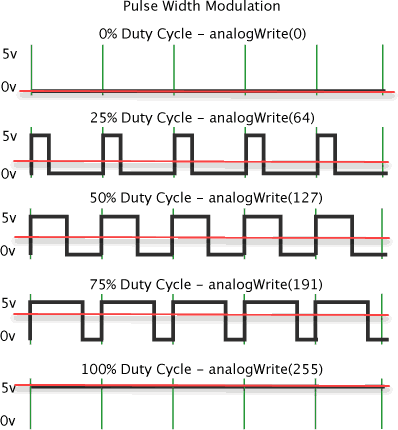Let us summarize this method with an example: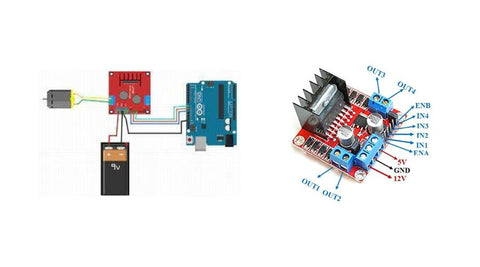In this example, we have
DC motor: Converts electrical energy to mechanical or rotational energy. 9V battery: To give power supply to the motor
Arduino Uno: To generate PWM signal to control the speed. L298N motor driver board: To drive the motor.

In this example, as shown in the image, Arduino generates a PWM signal to the motor driver through the command analogWrite(enA, value); which controls the speed of the motor. By changing the parameter ‘value’ we can change the speed of the motor. Thereby achieving the speed control of the dc motor.

### 1. What is the speed control of a DC motor?

Changing or increasing/decreasing the rate at which the motor is running by varying different parameters (armature resistance, flux, voltage) is called speed control of the DC motor.

### 2. Why do we control the speed of the DC motor?

Speed control of DC motor is required in various applications (like in robots or drones) to prevent mishaps.

### 3. How speed control of DC motor can be achieved?

Speed control of DC motor can be achieved by flux control, armature control, or voltage control. Voltage control is the most widely used method.

### 1. What is the speed control of a DC motor?

Changing or increasing/decreasing the rate at which the motor is running by varying different parameters (armature resistance, flux, voltage) is called speed control of the DC motor.

### 2. Why do we control the speed of the DC motor?

Speed control of DC motor is required in various applications (like in robots or drones) to prevent mishaps.

### 3. How speed control of DC motor can be achieved?

Speed control of DC motor can be achieved by flux control, armature control, or voltage control. Voltage control is the most widely used method.